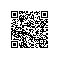# C技巧：结构体参数转成不定参数

#include <stdio.h>
#define func(...) myfunc((struct mystru){__VA_ARGS__})
struct mystru { const char *name; int number; };

void myfunc(struct mystru ms )
{
printf("%s: %d\n", ms.name ?: "untitled", ms.number);
}

int main(int argc, char **argv)
{
func("three", 3);
func("hello");
func(.name = "zero");
func(.number = argc, .name = "argc",);
func(.number = 42);
return 0;
}

three: 3
hello: 0
zero: 0
argc: 1
untitled: 42

myfunc((struct mystru){"three", 3});
myfunc((struct mystru){"hello"});
myfunc((struct mystru){.name = "zero"});
myfunc((struct mystru){.number = argc, .name = "argc",});
myfunc((struct mystru){.number = 42});使用钉钉扫一扫加入圈子
+ 订阅